Projective formalismNext: Perspective projection into two views Up: Mosaic Tutorial Previous: Mosaic Tutorial

Subsections

# Projective formalism

## Pinhole cameraThe pinhole camera is the most common camera model in Computer Vision.

We represent a pinhole camera by a plane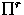called retinian plane and a point C called optical center. Let M be a 3D point with the coordinates (X Y Z T)t in the projective space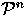.In figure 1 we show how we project the point M in m ( T u, T v,T), its image on the retinian plane. The formula 1 gives us the relation between M and m, P being the projection matrix. The projection matrix P can be decomposed into a product of three matrixes as we show in formula 2.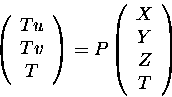(1)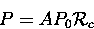(2)

where:

•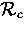, the 3D coordinates change matrix: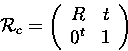(3)
• P0, the standard projection :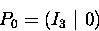(4)
• A, the 2D coordinates change matrix:(5)

The parameters of(i.e. rx,ry,rz,tx,ty,tz) are called the extrinsic parameters and the parameters of A (i.e.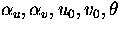) are called the intrinsic parameters. The parameter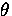(the angle between the rows and columns of the camera) is usually set to[Vai90].Next: Perspective projection into two views Up: Mosaic Tutorial Previous: Mosaic Tutorial